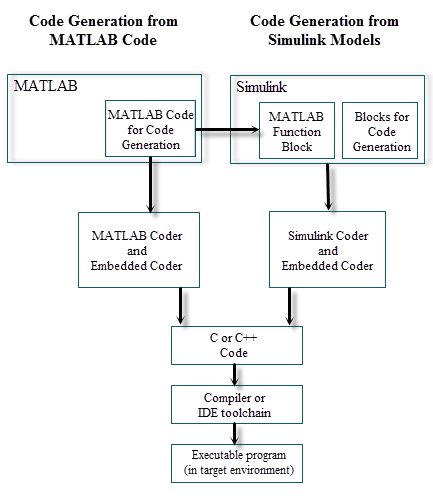Main Content

## Code Generation by Using Embedded Coder

### Code Generation Technology

MathWorks® code generation technology produces C or C++ code and executable programs for algorithms. You can write algorithms programmatically by using MATLAB® or graphically in the Simulink® environment. You can generate code for MATLAB functions and Simulink blocks that are useful for real-time and embedded applications. Generated source code and executable programs for floating-point algorithms match the functional behavior of MATLAB code execution and Simulink simulations to a high degree of fidelity. Using the Fixed-Point Designer product, you can generate fixed-point code that provides a bitwise match to model simulation results. Such broad support and high degree of accuracy are possible because code generation is tightly integrated with the MATLAB and Simulink execution and simulation engines. The built-in accelerated simulation modes in Simulink use code generation technology.

Code generation technology and related products provide tooling that you can apply to the V-model for system development. The V-model is a representation of system development that highlights verification and validation steps in the development process. For more information, see Validation and Verification for System Development.

To learn about model design patterns that include Simulink blocks, Stateflow® charts, and MATLAB functions, and map to commonly used C constructs, see Modeling Patterns for C Code.

### Code Generation Workflows with Embedded Coder

The Embedded Coder® product extends the MATLAB Coder™ and Simulink Coder products with features that you can use for embedded software development. Using the Embedded Coder product, you can generate code that has the clarity and efficiency of handwritten code. For example, you can:

• Generate code that is compact and fast, which is essential for real-time simulators, rapid prototyping boards, microprocessors used in mass production, and embedded systems.

• Customize the appearance of the generated code.

• Optimize generated code for a specific target environment.

• Integrate existing applications, functions, and data.

• Enable tracing, reporting, and testing options that facilitate code verification.

The code generator supports two workflows for designing, implementing, and verifying generated C or C++ code. The following figure shows the design and deployment environment options.Other products that support code generation, such as Stateflow software, are available.

To develop algorithms with MATLAB code for code generation, see Code Generation from MATLAB Code.

To implement algorithms as Simulink blocks and Stateflow charts in a Simulink model, and generate C or C++ code, see Code Generation from Simulink Models.

#### Code Generation from MATLAB Code

Code generation from the MATLAB code workflow with Embedded Coder requires the following products:

• MATLAB

• MATLAB Coder

• Embedded Coder

MATLAB Coder supports a subset of core MATLAB language features, including program control constructs, functions, and matrix operations. To generate C or C++ code, you can use MATLAB Coder projects or enter the function `codegen` in the MATLAB Command Window. Embedded Coder provides additional options and advanced optimizations for fine-grain control of generated code functions, files, and data. For more information about these options and optimizations , see Embedded Coder Capabilities for Code Generation from MATLAB Code.

For more information about generating code from MATLAB code, see Code Generation Workflow.

To get started generating code from MATLAB code using Embedded Coder, see Embedded Coder Capabilities for Code Generation from MATLAB Code.

#### Code Generation from Simulink Models

Code generation from the Simulink models workflow with Embedded Coder requires the following products:

• MATLAB

• MATLAB Coder

• Simulink

• Simulink Coder

• Embedded Coder

You can implement algorithms as Simulink blocks and Stateflow charts in a Simulink model. To generate C or C++ code from a Simulink model, Embedded Coder provides features for implementing, configuring, and verifying your model for code generation.

If you have algorithms written in MATLAB code, you can include the MATLAB code in a Simulink model or subsystem by using the MATLAB Function block. When you generate C or C++ code for a Simulink model, the MATLAB code in the MATLAB Function block is generated into C or C++ code and included in the generated source code.

To get started generating code from Simulink models using Embedded Coder, see Generate C Code from Simulink Models.

To learn how to model and generate code for commonly used C constructs using Simulink blocks, Stateflow charts, and MATLAB functions, see Modeling Patterns for C Code.

Download guide